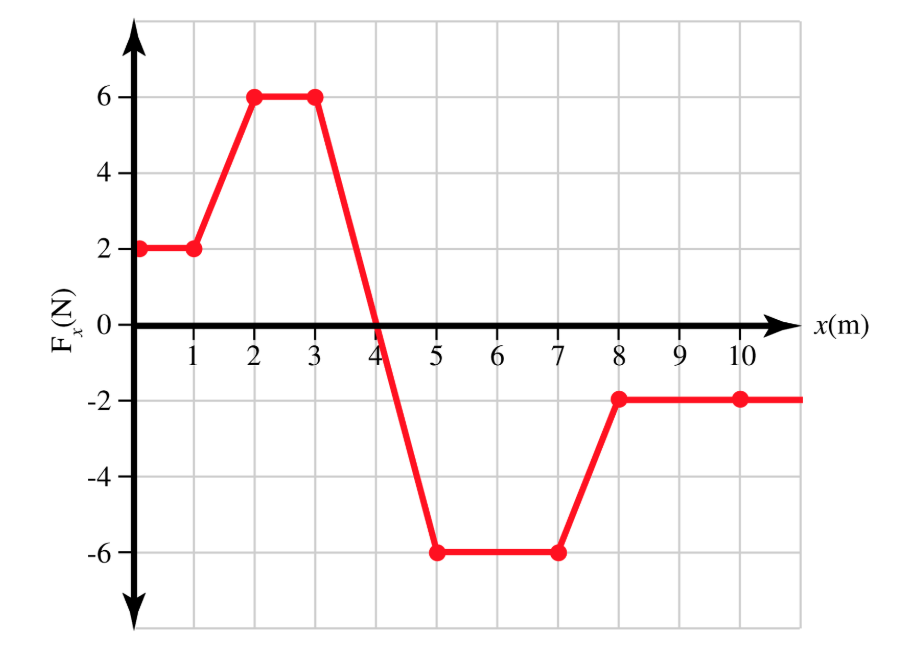64210x(m)923610-2-4-6EN(N)

Question

The graph shows the force acting on an object as a function of the position of the object. For each numbered interval given, find the work ??Wi done on the object. 1) from ?=0 mx=0 m to ?=2.90 m. 2) from ?=3.00 mx=3.00 m to ?=6.50 m. 3) from ?=7.00 mx=7.00 m to ?=9.50 m.help_outlineImage Transcriptionclose6 4 2 10 x(m) 9 2 3 6 10 -2 -4 -6 EN (N) fullscreen
Step 1
1. a) Work done is,
Step 2

Area under the Force-displacement graph will give the work done. Area from x=0 m to x=2.9 m is divided into three sections. First region is from x=0 m to x=1 m, second one is from x=1 m to x=2 m and third section is from x=2 m to x=2.9 m. Area or work done is,

Step 3

Want to see the full answer?

See Solution

Want to see this answer and more?

Our solutions are written by experts, many with advanced degrees, and available 24/7

See Solution
Tagged in

Work,Power and Energy